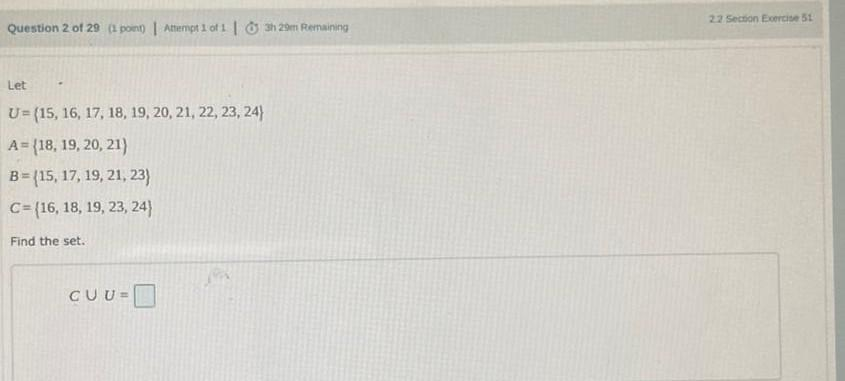Question:

# Let U=(15, 16, 17, 18, 19, 20, 21, 22, 23, 24) A={18, 19, 20, 21) B=(15, 17, 19, 21, 23) C=(16, 18, 19, 23, 24) Find the set. CυU=_____Let U=(15, 16, 17, 18, 19, 20, 21, 22, 23, 24) A={18, 19, 20, 21) B=(15, 17, 19, 21, 23) C=(16, 18, 19, 23, 24) Find the set. CυU=_____